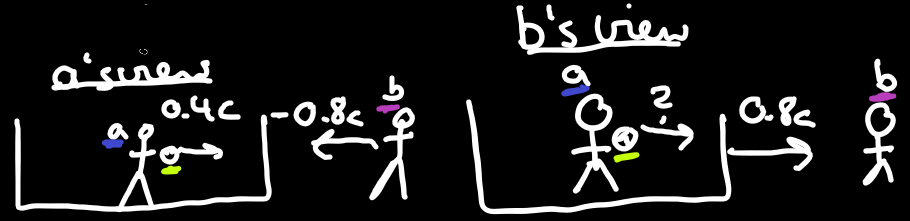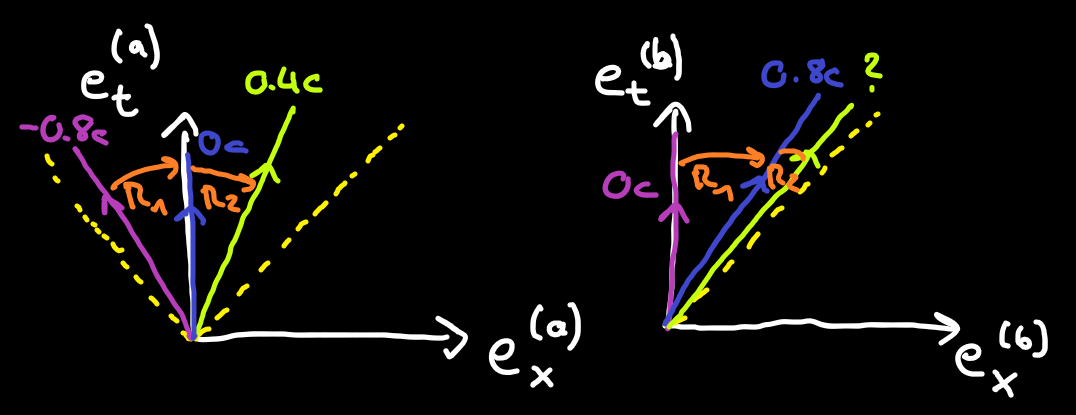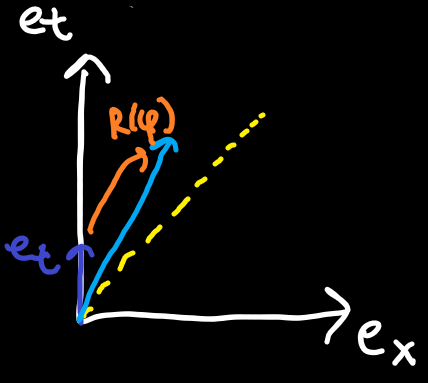### Special Relativity with Geometric Algebra - Spacetime Rotors

#### Velocity addition close to the speed of light

To motivate spacetime rotors, we need more trains (these are very popular in relativity apparently).Figure 1 - Left: Alice is on a train throwing a ball and sees Bob outside the train, Right: Bob looks at Alice throwing a ball in her moving train
Consider Alice is now on a train. On the train, she is throwing a ball with speed$0.4c$(yes, yes... very contrived). Outside of the train is Bob who can see the train moving at$0.8c$. Alice, on the train, sees herself standing still but sees Bob passing by her at$-0.8c$(ie. same speed but in the opposite direction of the train). We can now ask the question of how fast is the ball moving from the point of view of Bob.
If we just add up the speed of the train as seen by Bob and the speed of the ball as seen by Alice we get$0.8c+0.4c=1.2c$but this can't be as nothing can exceed the speed of light. Let's recall some things:
• From the paths section we know that the angle of paths is related to their speed
• We also know that resting observers have path velocities proportional to${e}_{t}$
• Claim: We can use our new rotors to rotate between path velocities to find the correct velocity from Bob's perspective
Okay this might sound far-fetched first. Rotate between spacetime velocities? Let's look at this sketch.Figure 2 - Diagonal yellow: Light. Left: Alice's view, Alice (blue) is at rest, Bob (purple) moves left, Ball (green) moves right. Right: Bob's view, Alice (blue) moves right, Bob (purple) is at rest, Ball (green) is moves right.
##### Alice's view (left)
Alice (blue) is at rest so her path velocity vector points in her${e}_{t}$direction. From Alice's view Bob (purple) is moving left so his velocity vector points left. The rotation from Bob's to Alice's velocity vector is done by applying the rotor${R}_{1}$to Bob's velocity vector. The ball moves to the right, so its velocity vector is rotated to the right. The rotation between Alice and the ball is done with the rotor${R}_{2}$.
The rotations between these vectors are all active transformations because the three velocity vectors are actually different from each other and it is not just Alice changing her perspective on a single unchanging vector.
##### Bob's view (right)
Bob's velocity vector (purple) is at rest, ie. points in his${e}_{t}$direction. Alice's velocity vector is rotated right with${R}_{1}$and the ball is rotated relative to Alice's velocity vector with${R}_{2}$.
##### Velocity Rotors
Now the key observation: the rotors in the left and the right diagram are identical. The values in them are the same (unlike for the vectors!). So if we know them in one diagram we can use them in the other. We know${R}_{1}$from both views, but we only know${R}_{2}$from Alice's view. But if we calcualte${R}_{2}$in Alice's view, we can just apply it to Alice's velocity vector in Bob's view.
So that's the idea. How to actually do this and how the angle relates to the speed comes in the next section.

#### Rotors in the Spacetime Algebra

To continue, we need to look at what the rotors in the Spacetime Algebra actually do. We will only need the rotors for bivectors squaring to$+1$, ie.${e}_{tx},{e}_{ty},{e}_{tz}$. Let's stick with${e}_{tx}$for now. How do we get rotors from bivectors? We stick them in the exponential function with an angle$\phi$, usually negated and halved because we need to apply a two-sided product.
$\begin{array}{}\text{(1)}& R\left(\phi \right)={e}^{-\frac{\phi }{2}{e}_{tx}}\end{array}$
Can we expand this further? Usually we get something involving sine and cosine for performing ordinary rotations, but that is because the bivector in the exponential squared to$-1$. It turns out in the case of$+1$we get hyperbolic sine and hyperbolic cosine. You can get this result by using the taylor series for the exponential function and comparing it with the taylor series of the hyperbolic sine and cosine functions. Anyway, we now get
$\begin{array}{}\text{(2)}& R\left(\phi \right)={e}^{-\frac{\phi }{2}{e}_{tx}}=\mathrm{cosh}\left(-\frac{\phi }{2}\right)+\mathrm{sinh}\left(-\frac{\phi }{2}\right){e}_{tx}\end{array}$
What does this rotor do? It's not ordinary rotation, it is hyperbolic rotation! What is hyperbolic rotation? I promised you earlier we could use these rotors to rotate velocity vectors and use them for velocity addition. Let's apply them to the rest velocity vector${e}_{t}$and see what we can infer from that
$\begin{array}{}\text{(3)}& R\left(\phi \right){e}_{t}\stackrel{~}{R}\left(\phi \right)=\mathrm{cosh}\left(\phi \right){e}_{t}+\mathrm{sinh}\left(\phi \right){e}_{x}\end{array}$
The steps between the left-hand side and right-hand side are left as an excercise. This will require some identities involving the hyperbolic sine and cosine functions, or you can just throw them into Wolfram Alpha to see what the parts simplify to.
We can see the result is some mix of${e}_{t}$and${e}_{x}$.Figure 3 - Yellow: Light. Dark blue: Velocity of object at rest. Light blue: Velocity of moving object. Orange: Rotor between object at rest and moving object.
To get some more intuition let's look at the slope of the result. The${e}_{t}$part is on the y-axis and the${e}_{x}$part is on the x-axis so we have
$\begin{array}{}\text{(4)}& slope\left(\phi \right)=\frac{\mathrm{sinh}\left(\phi \right)}{\mathrm{cosh}\left(\phi \right)}=\frac{1}{\mathrm{tanh}\phi }\end{array}$
So the slope is equal to one over the hyperbolic tangent$\mathrm{tanh}$. If you're familiar with neural networks you probably already know how the$\mathrm{tanh}$function looks (and perhaps other fields I'm not familiar with). Here's a sketchFigure 4 - Hyperbolic tangent function, zero at phi equal to 0, one towards positive and negative infinity.
The second part we need for plotting what the result looks like is the length of the resulting vector. The length in our graph is the euclidean length. This is of course not the length / distance we use in the algebra, but in our drawings, if the${e}_{x}$and${e}_{t}$components get bigger, then the vector we draw always gets bigger too. So we also have
$\begin{array}{}\text{(5)}& graphlength\left(\phi \right)=\sqrt{{\mathrm{cosh}}^{2}\left(\phi \right)+{\mathrm{sinh}}^{2}\left(\phi \right)}\end{array}$
Now we take a deeper look at the slope result we got earlier for different rotor angles$\phi$.
If$\phi =0$, then the slope is$\mathrm{\infty }$(vertical) and the graph length is$1$. This makes sense as rotating by$0$should leave the input unchanged. Furthermore, for$\phi \to \mathrm{\infty }$, we can see that$slope\to 1$. As we increase the angle of the rotor, the slope tends towards$1$. A slope of$1$is a 45° angle. Recall that light travels at 45° angles, so this is good! If we increase the angle, our velocity vector rotates towards the speed of light. Using these rotors we can never exceed (or even reach) 45°. Finally, the length also tends towards infinity, meaning if we rotate the rest velocity vector, its length will always increase.
##### Relation between velocity and angle
Given what we learnt, we can try to figure out the relationship between the angle$\phi$and the velocity$v$. We know that the slope for light is$1$, so$\mathrm{tanh}\left({\phi }_{light}\right)=1$. We also know that the rest velocity has slope infinity (vertical), ie.$\mathrm{tanh}\left(0\right)=0$. Based on these two data points, what do you think the relation is? The correct answer that also fits both data points is
$\begin{array}{}\text{(6)}& \mathrm{tanh}\left(\phi \right)=\frac{v}{c}\end{array}$
We will use this relation in the next section to finally solve our velocity addition problem.

#### Velocity addition close to the speed of light: revisitedFigure 5 - Diagonal yellow: Light. Left: Alice's view, Alice (blue) is at rest, Bob (purple) moves left, Ball (green) moves right. Right: Bob's view, Alice (blue) moves right, Bob (purple) is at rest, Ball (green) is moves right.
And we're back. This time equipped with the power of rotors that can rotate velocities and the relation between angles and velocities! We want to figure out the velocity of the ball from Bob's perspective (which we don't know yet). For that we look at Bob's frame where he is at rest and apply the two rotors (which we do know). Let me show you one more thing (that you probably already knew!) about rotors before we tackle the problem.
When we compose two rotors in the same plane we get (no matter what the bivector squares to)
$\begin{array}{}\text{(7)}& R\left({\phi }_{1}\right)R\left({\phi }_{2}\right)={e}^{-\frac{{\phi }_{1}}{2}{e}_{tx}}{e}^{-\frac{{\phi }_{2}}{2}{e}_{tx}}={e}^{-\frac{{\phi }_{1}+{\phi }_{2}}{2}{e}_{tx}}=R\left({\phi }_{1}+{\phi }_{2}\right)\end{array}$
When composing two rotations the angles just add. This is still the case even with our hyperbolic rotations. It turns out to solve the problems we don't even need to use rotors directly. First we calculate the two angles corresponding to the two velocities we were given. To get the angle from velocity we solve$\text{(6)}$for$v$. Then we add the angles. Finally we need to convert the resulting angle back to a velocity using$\text{(6)}$, and that will be the velocity of the ball as seen from Bob.
$\begin{array}{}\text{(8)}& {v}_{ball}=c\cdot \mathrm{tanh}\left({\phi }_{1}+{\phi }_{2}\right)=c\cdot \mathrm{tanh}\left({\mathrm{tanh}}^{-1}\left(0.8\right)+{\mathrm{tanh}}^{-1}\left(0.4\right)\right)\approx 0.9c\end{array}$
The ball moves at around$0.9c$from Bob's perspective.

#### Conclusion

We started by noticing that adding velocities close to the speed of light fails with ordinary addition because the speed of light can be exceeded. We then saw how we can relate velocity vectors using rotors with bivectors that square to$+1$. We noticed that these rotors rotate velocity vectors towards the speed of light but never exceed it. We also saw that the rotors don't change with passive transformations so we could compose them to solve our velocity addition problem. The result was that we can add the rotor angles for rotors in the same plane and relate them to velocities using the formula$\mathrm{tanh}\left(\phi \right)=\frac{v}{c}$.

#### Formulas

• Relation between angle and velocity:$\mathrm{tanh}\left(\phi \right)=\frac{v}{c}$
• Addition of angles:${\phi }_{a+b}={\phi }_{a}+{\phi }_{b}$
• Addition of velocities:${v}_{a+b}=c\cdot \mathrm{tanh}\left({\phi }_{a+b}\right)=c\cdot \mathrm{tanh}\left({\mathrm{tanh}}^{-1}\left(\frac{{v}_{a}}{c}\right)+{\mathrm{tanh}}^{-1}\left(\frac{{v}_{b}}{c}\right)\right)$

#### Up next

Next we will look at how we can take measurements in Spacetime.